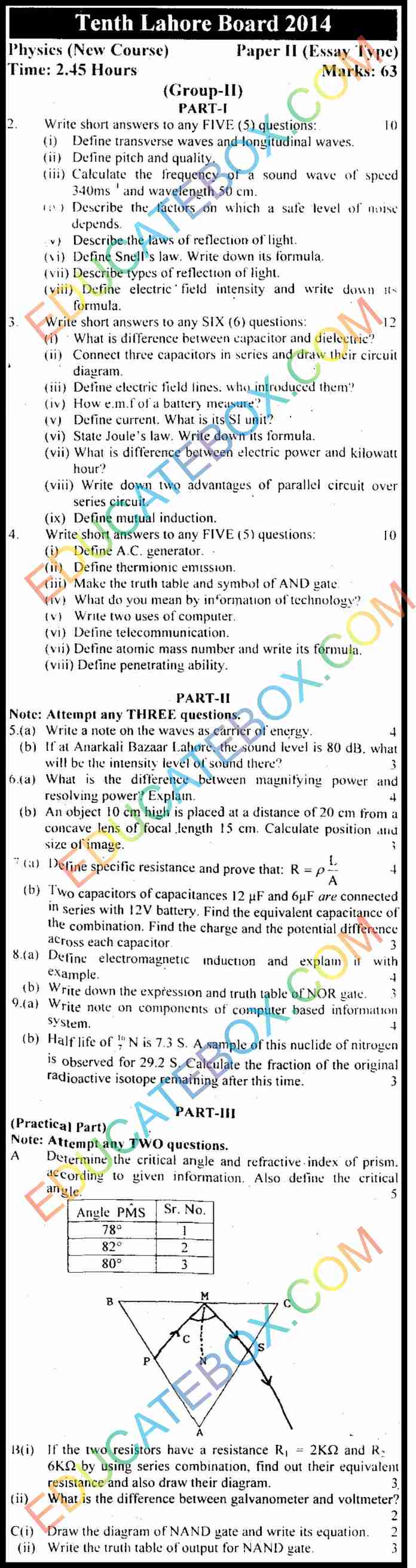# Past Paper 10th Class Physics Lahore Board 2014 English Medium Group2

0
304

## Past Paper 10th Class Physics Lahore Board 2014 English Medium Group 2

Physics paper of 10th class Lahore board 2014 of group 1 consists of 12 multiple choice questions, 3 subjective questions having 8 short questions each, 5 long questions and 3 practical questions.  Now there is no more separate practical paper for science subjects. The practical part is included in subjective part of the paper.

Some of the important multiple choice questions are as follows:

• What is an example of shock absorber of the vehicles?
• A large ripple tank with a vibrator working at a frequency of 30 Hz. produces 25 complete waves in a distance of 50 cm. What is the velocity of the wave?
• For a normal person, audible frequency range for sound wave lies between what?
• An object is 14 cm in front of a concave mirror. The image is 5.8 cm behind the mirror. What is the focal length of the mirror?
• What is the power rating of a lamp connected to a 12V source when it carries a current of 2.5 A?
• What is speed of sound in air?
• What does information mean in computer terminology?

Objective Type

## Past Paper Class 10 Physics Lahore Board 2014 English Medium Group 2 – Subjective Type

Some of the subjective short questions are as follows:

• Define transverse waves and longitudinal waves
• Define pitch and quality
• Calculate the frequency of a sound wave of speed 340ms and wavelength 50 cm.
• Describe the factors on which a safe level of noise depends
• Describe the laws of reflection of light?
• Define Snell’s law. Write down its formula.
• Define electric field intensity and write down its formula.
• Connect three capacitors in series and draw their circuit diagram
• Define electric field lines. Who introduced them?
• What is difference between electric power and kilowatt hour?
• Write down two advantages of parallel circuit over series circuit/
• Make the truth table and symbol of AND gate
• What do you mean by information of technology?
• Define atomic mass number and write its formula.
• An object 10 cm high is placed at a distance of 20 cm from a concave lens of focal length 15 cm. Calculate position and size of image.